# Recursion Tree | Solving Recurrence Relations

## Recursion Tree-

• Like Master’s Theorem, Recursion Tree is another method for solving the recurrence relations.
• A recursion tree is a tree where each node represents the cost of a certain recursive sub-problem.
• We sum up the values in each node to get the cost of the entire algorithm.

## Steps to Solve Recurrence Relations Using Recursion Tree Method-

### Step-01:

Draw a recursion tree based on the given recurrence relation.

### Step-02:

Determine-

• Cost of each level
• Total number of levels in the recursion tree
• Number of nodes in the last level
• Cost of the last level

### Step-03:

Add cost of all the levels of the recursion tree and simplify the expression so obtained in terms of asymptotic notation.

Following problems clearly illustrates how to apply these steps.

## Problem-01:

Solve the following recurrence relation using recursion tree method-

T(n) = 2T(n/2) + n

## Solution-

### Step-01:

Draw a recursion tree based on the given recurrence relation.

The given recurrence relation shows-

• A problem of size n will get divided into 2 sub-problems of size n/2.
• Then, each sub-problem of size n/2 will get divided into 2 sub-problems of size n/4 and so on.
• At the bottom most layer, the size of sub-problems will reduce to 1.

This is illustrated through following recursion tree-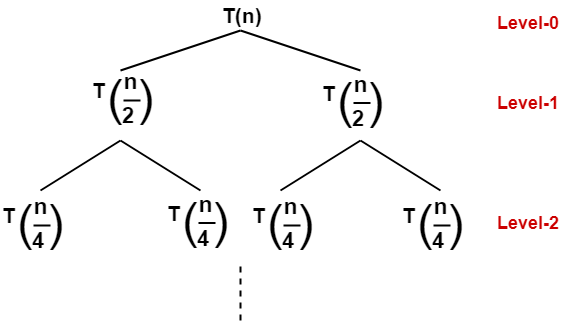The given recurrence relation shows-

• The cost of dividing a problem of size n into its 2 sub-problems and then combining its solution is n.
• The cost of dividing a problem of size n/2 into its 2 sub-problems and then combining its solution is n/2 and so on.

This is illustrated through following recursion tree where each node represents the cost of the corresponding sub-problem-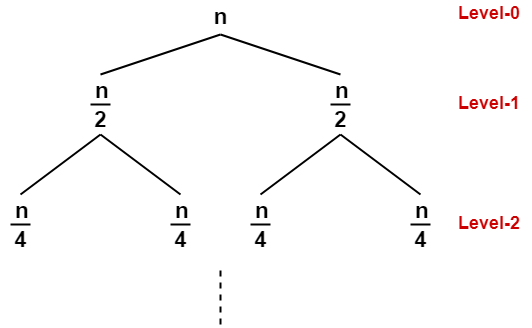### Step-02:

Determine cost of each level-

• Cost of level-0 = n
• Cost of level-1 = n/2 + n/2 = n
• Cost of level-2 = n/4 + n/4 + n/4 + n/4 = n and so on.

### Step-03:

Determine total number of levels in the recursion tree-

• Size of sub-problem at level-0 = n/20
• Size of sub-problem at level-1 = n/21
• Size of sub-problem at level-2 = n/22

Continuing in similar manner, we have-

Size of sub-problem at level-i = n/2i

Suppose at level-x (last level), size of sub-problem becomes 1. Then-

n / 2x = 1

2x = n

Taking log on both sides, we get-

xlog2 = logn

x = log2n

∴ Total number of levels in the recursion tree = log2n + 1

### Step-04:

Determine number of nodes in the last level-

• Level-0 has 20 nodes i.e. 1 node
• Level-1 has 21 nodes i.e. 2 nodes
• Level-2 has 22 nodes i.e. 4 nodes

Continuing in similar manner, we have-

Level-log2n has 2log2n nodes i.e. n nodes

### Step-05:

Determine cost of last level-

Cost of last level = n x T(1) = θ(n)

### Step-06:

Add costs of all the levels of the recursion tree and simplify the expression so obtained in terms of asymptotic notation-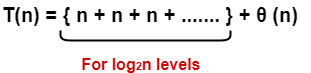= n x log2n + θ (n)

= nlog2n + θ (n)

= θ (nlog2n)

## Problem-02:

Solve the following recurrence relation using recursion tree method-

T(n) = T(n/5) + T(4n/5) + n

### Step-01:

Draw a recursion tree based on the given recurrence relation.

The given recurrence relation shows-

• A problem of size n will get divided into 2 sub-problems- one of size n/5 and another of size 4n/5.
• Then, sub-problem of size n/5 will get divided into 2 sub-problems- one of size n/52 and another of size 4n/52.
• On the other side, sub-problem of size 4n/5 will get divided into 2 sub-problems- one of size 4n/52 and another of size 42n/5and so on.
• At the bottom most layer, the size of sub-problems will reduce to 1.

This is illustrated through following recursion tree-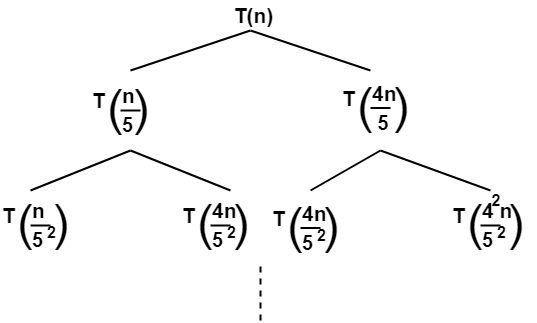The given recurrence relation shows-

• The cost of dividing a problem of size n into its 2 sub-problems and then combining its solution is n.
• The cost of dividing a problem of size n/5 into its 2 sub-problems and then combining its solution is n/5.
• The cost of dividing a problem of size 4n/5 into its 2 sub-problems and then combining its solution is 4n/5 and so on.

This is illustrated through following recursion tree where each node represents the cost of the corresponding sub-problem-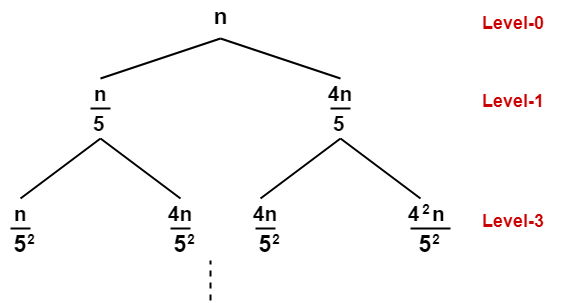### Step-02:

Determine cost of each level-

• Cost of level-0 = n
• Cost of level-1 = n/5 + 4n/5 = n
• Cost of level-2 = n/52 + 4n/52 + 4n/52 + 42n/52 = n

### Step-03:

Determine total number of levels in the recursion tree. We will consider the rightmost sub tree as it goes down to the deepest level-

• Size of sub-problem at level-0 = (4/5)0n
• Size of sub-problem at level-1 =(4/5)1n
• Size of sub-problem at level-2 =(4/5)2n

Continuing in similar manner, we have-

Size of sub-problem at level-i = (4/5)in

Suppose at level-x (last level), size of sub-problem becomes 1. Then-

(4/5)xn = 1

(4/5)x = 1/n

Taking log on both sides, we get-

xlog(4/5) = log(1/n)

x = log5/4n

∴ Total number of levels in the recursion tree = log5/4n + 1

### Step-04:

Determine number of nodes in the last level-

• Level-0 has 20 nodes i.e. 1 node
• Level-1 has 21 nodes i.e. 2 nodes
• Level-2 has 22 nodes i.e. 4 nodes

Continuing in similar manner, we have-

Level-log5/4n has 2log5/4n nodes

### Step-05:

Determine cost of last level-

Cost of last level = 2log5/4n x T(1) = θ(2log5/4n) = θ(nlog5/42)

### Step-06:

Add costs of all the levels of the recursion tree and simplify the expression so obtained in terms of asymptotic notation-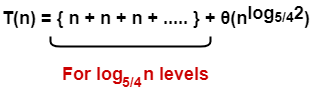= nlog5/4n + θ(nlog5/42)

= θ(nlog5/4n)

## Problem-03:

Solve the following recurrence relation using recursion tree method-

T(n) = 3T(n/4) + cn2

### Step-01:

Draw a recursion tree based on the given recurrence relation-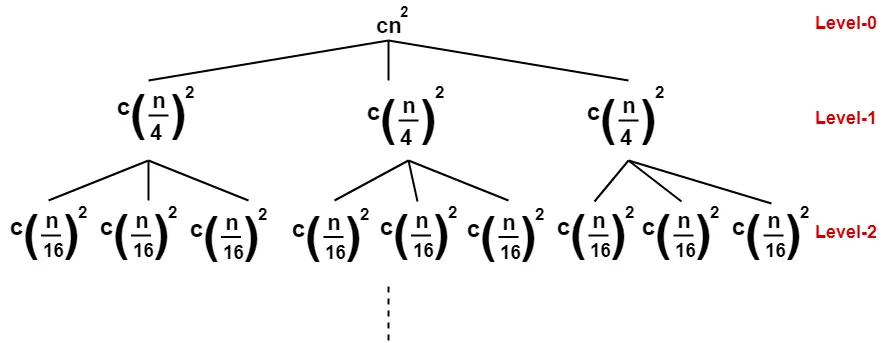(Here, we have directly drawn a recursion tree representing the cost of sub problems)

### Step-02:

Determine cost of each level-

• Cost of level-0 = cn2
• Cost of level-1 = c(n/4)2 + c(n/4)+ c(n/4)2 = (3/16)cn2
• Cost of level-2 = c(n/16)2 x 9 = (9/162)cn2

### Step-03:

Determine total number of levels in the recursion tree-

• Size of sub-problem at level-0 = n/40
• Size of sub-problem at level-1 = n/41
• Size of sub-problem at level-2 = n/42

Continuing in similar manner, we have-

Size of sub-problem at level-i = n/4i

Suppose at level-x (last level), size of sub-problem becomes 1. Then-

n/4x = 1

4x = n

Taking log on both sides, we get-

xlog4 = logn

x = log4n

∴ Total number of levels in the recursion tree = log4n + 1

### Step-04:

Determine number of nodes in the last level-

• Level-0 has 30 nodes i.e. 1 node
• Level-1 has 31 nodes i.e. 3 nodes
• Level-2 has 32 nodes i.e. 9 nodes

Continuing in similar manner, we have-

Level-log4n has 3log4n nodes i.e. nlog43 nodes

### Step-05:

Determine cost of last level-

Cost of last level = nlog43 x T(1) = θ(nlog43)

### Step-06:

Add costs of all the levels of the recursion tree and simplify the expression so obtained in terms of asymptotic notation-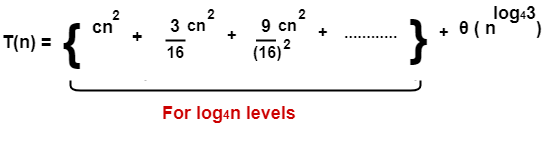= cn2 { 1 + (3/16) + (3/16)2 + ……… } + θ(nlog43)

Now, { 1 + (3/16) + (3/16)2 + ……… } forms an infinite Geometric progression.

On solving, we get-

= (16/13)cn2 { 1 – (3/16)log4n } + θ(nlog43)

= (16/13)cn2 – (16/13)cn2 (3/16)log4n + θ(nlog43)

= O(n2)

To gain better understanding about Recursion Tree,

Watch this Video Lecture

Next Article- DFS Algorithm

Get more notes and other study material of Design and Analysis of Algorithms.

Watch video lectures by visiting our YouTube channel LearnVidFun.

SummaryArticle Name
Recursion Tree | Solving Recurrence Relations
Description
Like Master's theorem, recursion tree method is another method for solving recurrence relations. A recursion tree is a tree where each node represents the cost of a certain recursive sub-problem. We will follow the following steps for solving recurrence relations using recursion tree method.
Author
Publisher Name
Gate Vidyalay
Publisher Logo